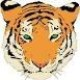Zum Hauptinhalt gehen

### Suche### Suche## Kommentare

1 Kommentar

•###### Peter Thane

Where does the date come from? If it is always the date of printing then you could just add on field with 7 sub strings in it and then Use VB to pick out the bits you want:In order the VB needed would be

String 1: (YY..)

Value = Now()

Value = Year(Value)

Value = right(Value,4)

Value = Left(Value,2)

String 2 (d.)

Value = Now()

Value = Day(Now)

If Value < 10 then

Value = 0

else Value = Left(Value,1)

end if

Value = Value

String 3 (M.)

Value = Now()

Value = Month(Value)

If Value < 10 then

Value = 0

else Value = Left(Value,1)

end if

Value = Value

String 4 (.d)

Value = Now()

Value = Day(Now)

Value = Right(Value,1)

String 5 (.M)

Value = Now()

Value = Month(Value)

Value = Right(Value,1)

String 6 (..YY)

Value = Now()

Value = Year(Value)

Value = right(Value,2)

Bitte melden Sie sich an, um einen Kommentar zu hinterlassen.